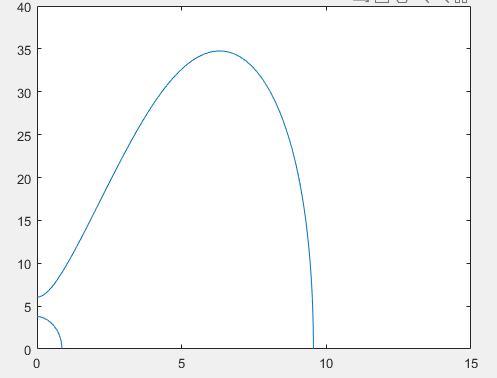搜索内容 搜索用户

# [未答复] 隐函数作图发现有多条曲线，怎么分别对曲线求定积分？kkkMONred 发表于 2022-8-4 14:15:09
10 财富积分

 本帖最后由 kkkMONred 于 2022-8-4 14:29 编辑   本人新手+菜鸟一枚，遇到了点问题实在想不出怎么解决，求论坛大神来帮忙解决。   问题：有一个比较复杂的隐函数f（x，y），我用fimplicit可以看到函数的图像分布，重点是这个函数有两条甚至三条曲线（取决于函数里的一个常数v的大小，但两条或三条不关键），关键是怎么分别求这些曲线x轴的积分（也就是曲线面积。要分别求，且上面条曲线的面积也是对x轴，并不要减去下面的小曲线面积）？   情况如下：1. 比如当我将函数里的常数设置为 v=0.5 时，用fimplicit画出f（x，y）的图像如下；                         2. 曲线都有值，可以用 “x=fig.XData”，“y=fig.YData”将值取出赋给x和y                         3. 但在用integral准备求积分时，会弹出“第一个输入必须包含唯一的值”的错误信息代码如下： v=0.5; %关键调整常数 h = 1.47;  %其他常数 R = 76.2; B = h^2/(12*R^2); NU = 0.33; l = 22; syms x y p0 = 0.5*(1-NU)*( (1-NU^2)*(y*pi/l)^4 + B*(x^2+(y*pi/l)^2)^4 ) + 0.5*B*(1-NU)*( 2*(2-NU)*(y*pi/l)^2*x^2 + x^4 - 2*NU*(y*pi/l)^6 - 6*(y*pi/l)^4*x^2 - (4-NU)*(y*pi/l)^2*x^4 - 2*x^6 ); p2 = 0.5*(1-NU)*( (3+2*NU)*(y*pi/l)^2 +x^2 +(x^2+(y*pi/l)^2)^2 + ((3-NU)/(1-NU))*B*(x^2+(y*pi/l)^2)^3 ); p4 = 1 + 0.5*(3-NU)*(x^2+(y*pi/l)^2) + B*(x^2+(y*pi/l)^2)^2; fun = v^6 - p4*v^4 + p2*v^2 - p0; FUN = matlabFunction(fun); fig = fimplicit(FUN,[0 15 0 40]); 复制代码
 本版积分规则 回帖后跳转到最后一页
##### 热门教程